14

8

4

2

# ComplexPhasePortrait.jlThis package is a Julia implementation of the phase portrait ideas presented in Elias Wegert's book "Visual Complex Functions".

## Installation

From the Julia command prompt:

``````Pkg.clone("git://github.com/JuliaHolomorphic/ComplexPhasePortrait.jl.git")
``````

## Examples

There is so far one exported function, `portrait`, and here I will try to detail its use. First we need function data over a grid.

``````using ComplexPhasePortrait

nx = 1000
x = range(-1, stop=1, length=nx)
Z = x' .+ reverse(x)*im

f = z -> (z - 0.5im)^2 * (z + 0.5+0.5im)/z
fz = f.(Z)
``````

Now a basic phase plot.

``````img = portrait(fz)
``````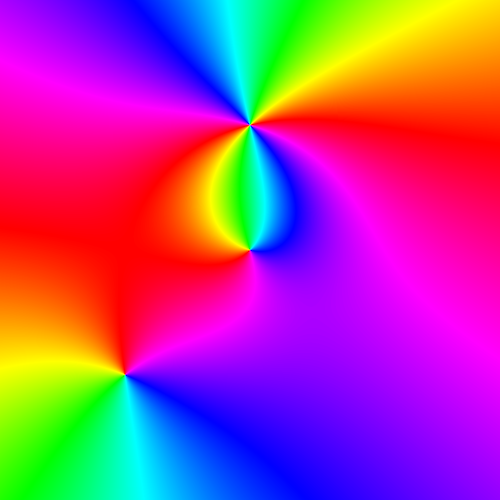Now for a basic plot using NIST coloring.

``````img = portrait(fz, ctype="nist")
``````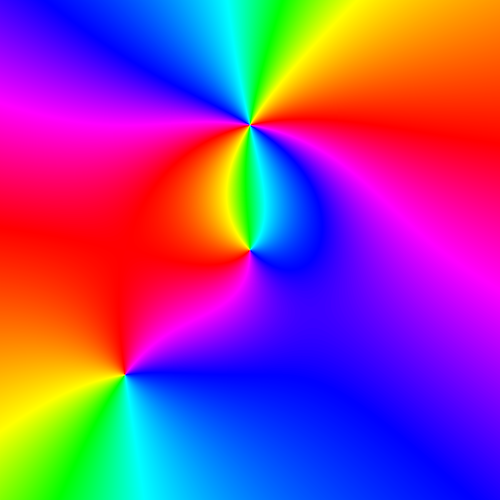Lines of constant phase are given by

``````img = portrait(fz, PTstepphase)
``````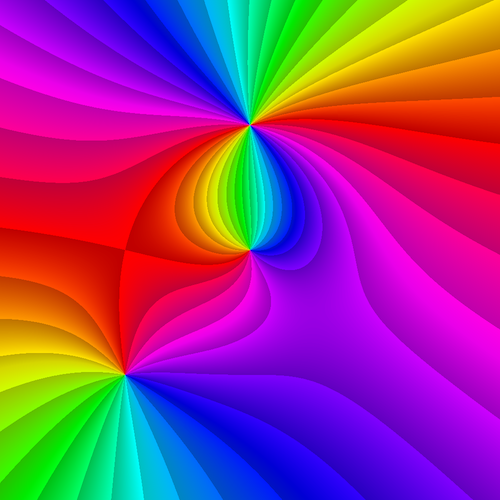Lines of constant modulus are given by

``````img = portrait(fz, PTstepmod)
``````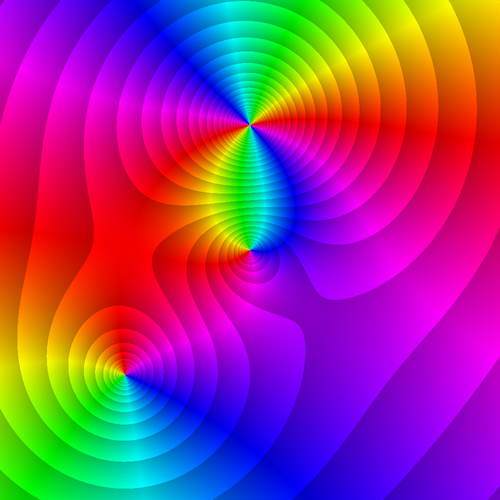Finally, a conformal grid is given by

``````img = portrait(fz, PTcgrid)
``````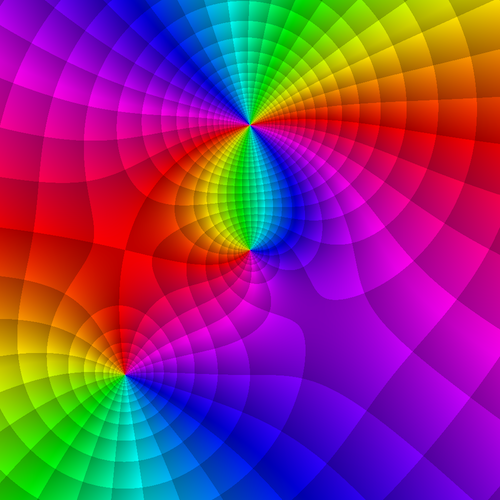10/04/2015

4 months ago

40 commits